Next: Memory Constraints Up: Theory: Optimization Methods Previous: Quasi-Newton (Variable Metric)

## Use of Second Derivatives : Levenberg-Marquardt

If we are able to efficiently calculate both the gradient,, and the Hessian matrix, A, at a given point a then we can use more efficient algorithms for locating the minima. In the above an iterative solution was required both because we were dealing with non-linear functions and in order to build up an estimate of the Hessian (either directly or indirectly). If we have a twice-differentiable analytic form for the function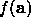we can generate the Hessian directly. The Levenberg-Marquardt (LM) Method uses a neat trick to take advantage of this. We saw above that if A is a good approximation to the actual form at the minimum (this is usually close to the true minimum) then we should update our current estimate of the minimum, a, using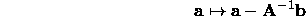However, if we are too far from the minimum A may not be a good approximation to the local shape of the function, and the best we can do is to move in a downhill directionThe LM algorithm combines these approaches in the following way: Define a new n x n matrix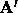from A as follows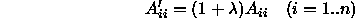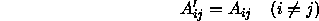Where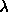is a regularization constant. At any point, a, the suggested next point is given by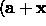) where x is the solution to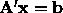. Whenis small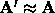, so we get a Newton-type jump toward the minima. Whenis largebecomes diagonally dominant and x gives a guess at a suitable downhill step.

We start with a modest value for, say 0.001, and evaluate. The algorithm then proceeds as follows:

• Solve for x and evaluate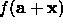• If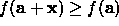increaseby a factor of 10.
• If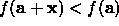decreaseby a factor of 10 and update the current solution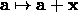The algorithm iterates until some convergence criteria are reached. Typically this means that we stop when successive improvements in the value ofonly change its value by some small fraction ( for instance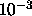).Next: Memory Constraints Up: Theory: Optimization Methods Previous: Quasi-Newton (Variable Metric)

Bob Fisher
Fri Mar 28 14:12:50 GMT 1997